# Programming with Python¶

## Contents:¶

1. Useful tools for data analysis

2. Case study: How warm was Europe in the past?

# Useful tools for data analysis¶

## Jupyter Notebook¶

Most data analysis needs to be accompanied by some form of reporting, explaining which data has been used, where the data comes from, which analysis method is being used and why, what are the conclusions of the analysis, and discussion. Jupyter Notebook is an open-source web application that allows us to create and share documents that contain live code, equations, visualizations and narrative text. If you have installed the Anaconda distribution on your computer, it should already be available. We can start Jupyter Notebook from the Anaconda Navigator or the command line.

Jupyter Notebooks contain both Python code and text, which is formatted in Markdown. Here are a few Markdown formats:

• Headings with #, ##, ###, ...
• Inline code enclosed with backticks like so: print('hello'), and code blocks with 
• Emphasis, aka italics, with *asterisks* or _underscores_
• Enumerated lists with 1., 2., etc., or bullet points with *
• Links with [I'm an inline-style link to Google](https://www.google.com)
• Images ![alt text](https://url/image.png "Logo Title Text 1")
• LaTex formulas with $ and $$ See Markdown Cheatsheet for more. ## JupterLab¶ Similar to Jupyter Notebook, but with many additional features focused on interactive, exploratory computing. The JupyterLab interface consists of a main work area containing tabs of documents and activities, a collapsible left sidebar, and a menu bar. The left sidebar contains a file browser, the list of running kernels and terminals, the command palette, the notebook cell tools inspector, and the tabs list. It is also good for viewing large CSV files. ## Python Data Analysis Library¶ The Python Data Analysis Library pandas provides high-performance, easy-to-use data structures and data analysis tools. Most importantly, it implements a fast and efficient DataFrame object for data manipulation with integrated indexing, and tools for reading and writing data between in-memory data structures and different formats. There's also a 10 minutes Pandas tutorial. Here we just show a quick example of pandas, using the data_reader package (which must be installed separately) to read and plot the daily low and high prices of the Amazon stock. In : import pandas_datareader.data as web import matplotlib.pyplot as plt import pandas as pd symbol = 'AMZN' # Amazon stock start = '2019-04-22' end = '2020-11-29' df = web.DataReader(name=symbol, data_source='yahoo', start=start, end=end) df.head()  Out: High Low Open Close Volume Adj Close Date 2019-04-22 1888.420044 1845.640015 1855.400024 1887.310059 3373800 1887.310059 2019-04-23 1929.260010 1889.579956 1891.199951 1923.770020 4640400 1923.770020 2019-04-24 1929.689941 1898.160034 1925.000000 1901.750000 3675800 1901.750000 2019-04-25 1922.449951 1900.310059 1917.000000 1902.250000 6099100 1902.250000 2019-04-26 1951.000000 1898.000000 1929.000000 1950.630005 8432600 1950.630005 In : %matplotlib inline import matplotlib.pylab as pylab pylab.rcParams['figure.figsize'] = 10, 7.5 ax = df[['Low','High']].plot();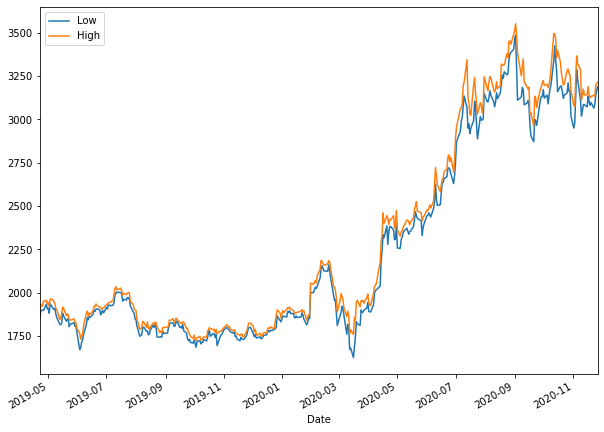# How do I become a data analyst?¶ The basic mathematical tools every data analyst needs to know are grounded in Linear Algebra, Optimisation, Statistics, and Probability Theory. Some of the third and fourth year courses that might be helpful are • MATH36001 - Matrix Analysis • MATH36061 - Convex Optimization • MATH38001 - Statistical Inference • MATH38141 - Regression Analysis • MATH38161 - Multivariate Statistics and Machine Learning • MATH38032 - Time Series Analysis • MATH46101 - Numerical Linear Algebra • MATH48091 - Statistical Computing Apart from being proficient in Python and the pandads package, a data analyst knows about various data analysis and machine learning techniques such as • statistical hypothesis testing • gradient descent methods • k-nearest neighbor clustering • simple, multiple, and logistic regression • decision trees • neural networks Many of these techniques are implemented in the Python package scikit-learn. Check out the extensive example collection. # How do I become a data scientist?¶ In addition to knowing data analysis techniques, a data scientist is able to write their own analysis algorithms and judge their suitability and reliability scientifically. A data scientist not only knows how to use advanced machine learning algorithms, but understands their internally workings and has read relevant scientific literature before using them. A good way to get familiar with the fundamental data science toos and algorithms is to code them from scratch, using only basic Python language. I strongly recommend the book Data Science from Scratch by Joel Grus (O'Reilly 2015). Indeed, a great deal of work can be done without using any of the above-mentioned libraries. We will best demonstrate this with a concrete data analysis problem. # Case study: How warm was Europe in the past?¶ Problems. Let us solve the following related problems: 1. What were the extreme average temperatures in the past 500 years in Europe? 2. How did the temperature change? 3. What did it look like at a certain point in time (a date or some approximant of that)? ## Loading the data¶ Obviously, Python itself does not provide the needed data. This is where searching the internet comes in handy, leading us to the historical paleoclimatological data of the NCDC (National Climatic Data Center). From their FTP site (ftp://ftp.ncdc.noaa.gov/pub/data/paleo/historical/) we can download various data. Some of the data, along with other info and the copyright notice, is available in the file europe-seasonal.txt (ftp://ftp.ncdc.noaa.gov/pub/data/paleo/historical/europe-seasonal.txt). The data itself looks like this: Year DJF MAM JJA SON Annual 1500 -0.945 7.157 17.483 8.990 8.166 1501 -0.850 7.435 17.401 8.687 8.163 1502 -1.053 6.872 17.906 9.071 8.194 ... 2002 0.207 9.214 18.905 9.301 9.508 2003 -1.101 8.521 19.615 9.838 9.374 2004 0.187 8.297 18.325 10.073 9.235 The column "year" holds the year of each row's data "DJF" stands for Winter (December, January, February), "MAM" stands for Spring (March, April, May), "JJA" stands for Summer (June, July, August), "SON" stands for Autumn (September, October, November), and "Annual" is the average temperature for the given year. We can copy just this part into a new file and save it under some name, for example "europe-seasonal.dat". Notice that this is not exactly a CSV file like we saw before, as the separators are strings of whitespaces of a varying length. The columns of this file are defined by their length (the year holds 4 characters and the rest hold 12 characters each). Luckily, this is not a problem: the split function that we used earlier uses exactly strings of whitespaces of a varying length as separators if it's not given a different one. So, one line from the above file can be split like this: year,djf,mam,jja,son,annual = line.strip().split()  The additional strip() call removes leading and trailing whitespaces (each line ends with a new-line characters that we want to remove). However, don't forget: each of the year, djf, mam, jja, son, and annual is a string now and needs to be converted to either int or float if we are to use it as such. A note on organizing our code. Given that we want to write several programs dealing with the same data, creating a module with some common functionality is a reasonable way to go. The first function to write would be the one fetching the data from the above file. There are two things to consider here: 1. What to return? We can write it to return either an iterator or a list of all values. Since this data set is not very big, the two approaches don't differ much. Still, iterator is usually a better option and we'll do that here. 2. How to store each year's (row's) data? Obvious choices are a tuple or a dictionary. The latter is a tad more descriptive, but a tuple is a bit easier to create, so we'll work with tuples. This is really just the matter of a personal choice. So, what we need to do is read the file line by line, split each line, convert the elements to int/float, put them in a tuple and yield them. Since we have created our own input file, we can choose the format. It will be as described above, but minus the header row, since we have no use for it. In other words, our file "europe-seasonal.dat" looks like this: 1500 -0.945 7.157 17.483 8.990 8.166 1501 -0.850 7.435 17.401 8.687 8.163 1502 -1.053 6.872 17.906 9.071 8.194 ... 2002 0.207 9.214 18.905 9.301 9.508 2003 -1.101 8.521 19.615 9.838 9.374 2004 0.187 8.297 18.325 10.073 9.235 We can now create a function that will read the data from this file: In : import os.path # This is the directory with the data data_dir = os.path.join("10a-temps", "eu-data") def seasonal_data(fname = os.path.join(data_dir, "europe-seasonal.dat")): """ Read the seasonal data from a fixed-width column file with the columns year, djf (Winter: December, January, February), mam (Spring: March, April, May), jja (Summer: June, July, August), son (Autumn: September, October, November), and annual (Annual average). The return value is an iterator that returns the tuple (year, djf, mam, jja, son, and annual) for each row of data. It is assumed that the file has no header. If the file does not exist, a FileNotFoundError exception is raised. """ with open(fname) as f: for line in f: fields = line.strip().split() # Extract year, convert it to int, join it back with # the rest of values converted to float, and yield # them all as a tuple # The try...except block ensures that the faulty data # (for example, a header) is ignored if len(fields) == 6: try: yield tuple([int(fields)] + [float(x) for x in fields[1:]]) except ValueError: continue  Notice the use of os.path.join above. While it will generally work to use "10a-temps/eu-data" instead of os.path.join("10a-temps", "eu-data"), there are situations when it will fail. A good program should always try to avoid those. More details can be found in this excellent explanation. Let us now get some basic info about the temperature in Europe in the past 500 years: In : # Get data to a list, as to avoid rereading the file several times. # We can afford this because the file is fairly small. data = list(seasonal_data()) # Minimums and maximums: print("The lowest average winter temperature: {:+7.3f}C".format(min(data, key=lambda t: t))) print(" This happened in the year {}.".format(min(data, key=lambda t: t))) print("The highest average winter temperature: {:+7.3f}C".format(max(data, key=lambda t: t))) print(" This happened in the year {}.".format(max(data, key=lambda t: t))) print("The lowest average summer temperature: {:+7.3f}C".format(min(data, key=lambda t: t))) print(" This happened in the year {}.".format(min(data, key=lambda t: t))) print("The highest average summer temperature: {:+7.3f}C".format(max(data, key=lambda t: t))) print(" This happened in the year {}.".format(max(data, key=lambda t: t))) min_annual = min(data, key=lambda t: t) max_annual = max(data, key=lambda t: t) print("The average anual temperature varied between {:+.3f}C in {} to {:+.3f}C in {}.".format( min_annual, min_annual, max_annual, max_annual ))  The lowest average winter temperature: -4.152C This happened in the year 1709. The highest average winter temperature: +1.734C This happened in the year 1990. The lowest average summer temperature: +16.477C This happened in the year 1902. The highest average summer temperature: +19.615C This happened in the year 2003. The average anual temperature varied between +7.006C in 1875 to +9.664C in 2000.  ## Plotting the temperature trend¶ The above analysis gave us a glimpse of what was going on with our temperature in the second half of the past millennium. However, to truly see what was going on, we should plot this data. First some setting up for this document: In : %matplotlib inline import matplotlib.pylab as pylab pylab.rcParams['figure.figsize'] = 10, 7.5  Note that %matplotlib inline is NOT a Python construct. It's purpose is to tell IPython Notebook (in which these notes are written) to include the plot in the document itself. As for the remaining two lines, they establish the size of the plot and can be used in Python as well. However, there are usually better ways to do it and this is used merely to set the default values for all the plots produced by the program. We are now ready to do some basic plotting: In : import matplotlib.pyplot as plt years = list() djfs = list() mams = list() jjas = list() sons = list() annuals = list() # There are more Pythonic ways to do this, for example with # NumPy's transposing or generator expressions, but this is # more straightforward. for year,djf,mam,jja,son,annual in seasonal_data(): years.append(year) djfs.append(djf) mams.append(mam) jjas.append(jja) sons.append(son) annuals.append(annual) plt.plot( years, djfs, "blue", years, mams, "green", years, jjas, "red", years, sons, "orange", years, annuals, "gray" ) plt.show()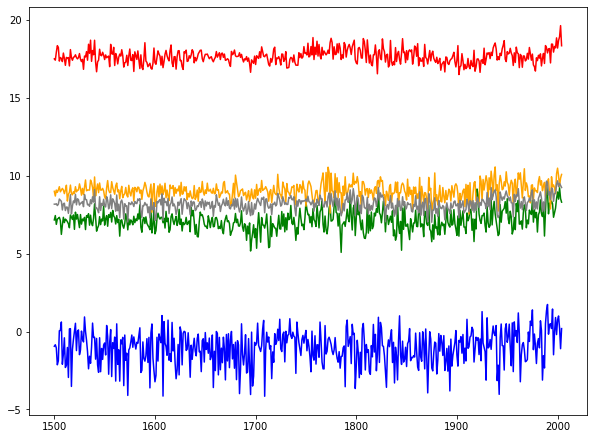So, how does this work? 1. import matplotlib.pyplot as plt imports the basic plotting module. 2. The function plot takes each dataset as a pair of two lists: x-values and y-values. Therefore, we create the lists of all the years and Spring/Summer/Autumn/Winter/annual temperatures in a very simple for loop. 3. We plot each of the lists of values, using years as the common list of values for the x-axis. 4. Finally, plt.show() tells the system that the plot is ready to be shown. From the above plot, we can observe several things: 1. Winters are always colder than Springs, which are usually (a bit) colder than Autumns, which are always colder than Summers. Average, as its name suggests, is in the middle. None of this is really surprising. 2. The average temperature varies more for the Winters than for other seasons. 3. Springs are varying more since around the beginning of the 19th century. What we cannot see are trends. For example, is the temperature rising? The above numbers suggest that Europe is warming up, because the maximum temperatures in Winter, Summer, and on average have all occured in recent years. However, these are just extremes that may or may not correlate with the general behaviour of the temperature. To observe that, we use smoothing. There are many different smoothing algorithms. Here, we shall use the moving average in its most simple form. If the temperature for a certain season in year y is given by the variable L_y, we create new variables:$$L'_y := \frac{1}{2r+1} \sum_{k=y-r}^{y+r} L_k,$$i.e.,$L'_y$is the average value of the temperatures from the year$y-r$up to (and including) the year$y+r$, where$r$(the radius) is some given number. The bigger the$r$, the smoother the result. So, how do we smooth a list in Python? Let us smooth only one element first, the$i$-th one. This means computing the sum$\sum_{k=i-r}^{i+r} L_k$and dividing it by$2r+1$. This means we need to: • get a part of the list: L[i-r:i+r+1] (the +1 part is here because the right limit is not included as a part of the new list), • find its sum:$\sum_{k=i-r}^{i+r} L_k =$sum(L[i-r:i+r+1]), • divide it with 2*r+1:$\frac{1}{2r+1} \sum_{k=i-r}^{i+r} L_k =$sum(L[i-r:i+r+1]) / (2*r+1). Repeating the above for all viable indices i can be easily done as a list comprehension: [ sum(L[i-r:i+r+1])/(2*r+1) for i in range(r, len(L)-r) ]  Finally, since we want to do this for a whole list, it is wise to compute 2*r+1 ahead and just store it in some variable. In : def smooth(L, r): """ Return a new list obtained from a list L by smoothing its values for a radius r. The returned list is shorter than L by 2*r elements because the border values are not smoothed. """ tot = 2*r + 1 return [ sum(L[i-r:i+r+1])/tot for i in range(r, len(L)-r) ]  Now, the smoothed versions of our temperature lists are easy to obtain: In : sdjfs = smooth(djfs, 5) # Display the first 3 and the last 3 elements of this new list print("Smoothed Winter temperatures: {}, ..., {}".format( ", ".join("{:.3}".format(x) for x in sdjfs[:3]), ", ".join("{:.3}".format(x) for x in sdjfs[-3:]) ))  Smoothed Winter temperatures: -0.917, -1.04, -1.16, ..., 0.338, 0.197, 0.169  Recall that the smoothed arrays are shorter than the original ones. This means that the years list is no longer appropriate for the x-axis and we need to create a new one, with the first and the last r elements removed: In : r = 5 sdjfs = smooth(djfs, r) print("len(smooth_djfs) = ", len(sdjfs)) print("len(years) = ", len(years)) syears = years[r:-r] print("len(smooth_years) =", len(syears))  len(smooth_djfs) = 495 len(years) = 505 len(smooth_years) = 495  As far as smoothing is concerned, this is it. However, there are various improvements that can be done to our plot. First, to make it easier to make some improvements, we take the figure and the subplot reference in two variables: fig = plt.figure() ax = plt.subplot(111)  This allows us to do the customisations that are related to them, and not just the plots themselves. For example: box = ax.get_position() ax.set_position([box.x0, box.y0, box.width * 0.8, box.height])  is used to reduce the width of the plotting area by 20% (to 0.8 of its original width), leaving some space on the right side for the legend. The legend itself is added by the legend function: plt.legend(bbox_to_anchor=(1.03, 1), loc="upper left", borderaxespad=0)  The description of the arguments used can be found in the function's reference. So, how does the legend get the names of the plots? This can be done in several different ways, the easiest one being the plot command itself. To do that, we draw all the plots one by one: plt.plot(syears, smooth(djfs, r), color="blue", label="Winter") plt.plot(syears, smooth(mams, r), color="green", label="Spring") ...  The value of the label argument is used as a description of the plot in the legend. We can also add titles to the plot and to its axes: plt.title("Smoothed temperatures through the century") plt.xlabel("Year") plt.ylabel("Temp (C)")  A grid is also trivial to add: plt.grid()  Notice how our plot has a big empty space on the right side. This is because the Matplotlib's automation decided that 2100 is a good right limit for the x-axis. However, we might want to use a different value, maybe 2015. We set this by calling the axis function: plt.axis([1500, 2015, -10, 35])  This sets the x-axis to display the values from 1500 to 2015, and the y-axis to display the values from -10 to 35. Of course, it would be better to derive these limits from the data. Luckily, we know that all the elements of djf (the Winter temperatures) are smaller than all the elements of the remaining lists; also, all the ellements of jja (the Summer temperatures) are bigger than all the elements of the remaining lists. This simplifies finding minimum and maximum, so our limits can be: plt.axis( [ years, years[-1], min(djfs), max(jjas) ] )  Finally, nothing bad will happen if we go a bit wider with the temperatures, i.e., if instead of the interval$[-4.152, 19.615]$we plot$[-5, 20]$. This can be done by some rounding magic, for example to the next value divisable by 5: plt.axis( [ years, years[-1], 5*floor(min(djfs)/5), 5*ceil(max(jjas)/5) ] )  This will add only a minor extra empty space to the top and to the bottom of our plot, but nothing big like the year 2100 added to the right. At the same time, our y-axis labels will turn out nicer. Instead of just showing it on the screen, we can also save the created plot: plt.savefig("europe-temps-smooth.png", bbox_inches="tight", dpi=200)  The bbox_inches defines the padding around the image, while the dpi argument stands for "Dots Per Inch". The bigger the value, the bigger the produced image. You can find these and other parameters in the documentation of the savefig function. Using what we've seen so far, we can produce the following plot: In : import matplotlib.pyplot as plt from math import floor, ceil r = 17 years = list() djfs = list() mams = list() jjas = list() sons = list() annuals = list() fig = plt.figure() ax = plt.subplot(111) # There are more Pythonic ways to do this, for example with # NumPy's transposing or generator expressions, but this is # more straightforward. for year,djf,mam,jja,son,annual in seasonal_data(): years.append(year) djfs.append(djf) mams.append(mam) jjas.append(jja) sons.append(son) annuals.append(annual) # Remove the first and the last r years as they cannot be properly smoothed syears = years[r:-r] # Compute the smoothed values plt.plot(syears, smooth(djfs, r), color="blue", label="Winter") plt.plot(syears, smooth(mams, r), color="green", label="Spring") plt.plot(syears, smooth(jjas, r), color="red", label="Summer") plt.plot(syears, smooth(sons, r), color="orange", label="Autumn") plt.plot(syears, smooth(annuals, r), color="gray", label="Average") # Shrink the plot box = ax.get_position() ax.set_position([box.x0, box.y0, box.width * 0.8, box.height]) # Add the legend plt.legend(bbox_to_anchor=(1.03, 1), loc="upper left", borderaxespad=0) # Define the axes limits plt.axis([years, years[-1], 5*floor(min(djfs)/5), 5*ceil(max(jjas)/5)]) # Title and axes labels plt.title("Smoothed temperatures through the century") plt.xlabel("Year") plt.ylabel("Temp (C)") # Display grid plt.grid() # Save the plot as a PNG image plt.savefig("europe-temps-smooth.png", bbox_inches="tight", dpi=200) # Show the plot plt.show()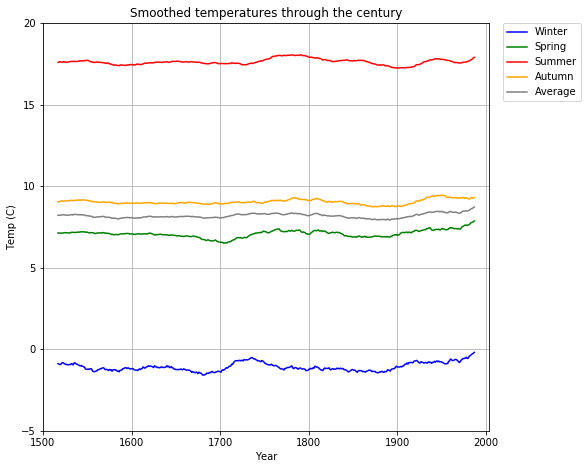Now, this is a much better presentation of the general temperature behaviour in Europe in the past 500 years. It would be nice to have this plot and some form of the previous one together, overlapping. Or, even better, have a several smoothed versions (for different values of r), in a way that the less smoothed ones are less visible, yet still present. We can do this by plotting as we did above, for several different values of r. The only question is how to achieve "less visibility" of certain plots. Those familiar with image processing probably know what an alpha-channel is. It holds an additional pixel information, not unlike color, that defines transparency of the pixel. The value can be any real number between$0$and$1$, where$0$means invisible and$1\$ means completely visible.

We shall define our alpha according to r, with some tweaking to make the final image look better:

In :
import matplotlib.pyplot as plt
from math import floor, ceil

# Radii for which to do the smoothing (0 = no smoothing)
rs = [ 0, 1, 3, 11, 17 ]

years = list()
djfs = list()
mams = list()
jjas = list()
sons = list()
annuals = list()

fig = plt.figure()
ax = plt.subplot(111)

# There are more Pythonic ways to do this, for example with
# NumPy's transposing or generator expressions, but this is
# more straightforward.
for year,djf,mam,jja,son,annual in seasonal_data():
years.append(year)
djfs.append(djf)
mams.append(mam)
jjas.append(jja)
sons.append(son)
annuals.append(annual)

# Get the smoothed amounts (for each point take the average of
# r values to the left and to the right
for r in rs:
# Remove the first and the last r years as they cannot  be properly smoothed
syears = years[r:-r] if r else years
# Compute the smoothed values
alpha = 0.1 + 0.9*(r/rs[-1])**2 if r else 0.1  # 0 = invisible, 1 = fully visible
plt.plot(syears, smooth(djfs, r), color="blue", alpha=alpha, label="Winter")
plt.plot(syears, smooth(mams, r), color="green", alpha=alpha, label="Spring")
plt.plot(syears, smooth(jjas, r), color="red", alpha=alpha, label="Summer")
plt.plot(syears, smooth(sons, r), color="orange", alpha=alpha, label="Autumn")
plt.plot(syears, smooth(annuals, r), color="gray", alpha=alpha, label="Average")

# Shrink the plot
box = ax.get_position()
ax.set_position([box.x0, box.y0, box.width * 0.8, box.height])

# Define the axes limits
plt.axis([years, years[-1], 5*floor(min(djfs)/5), 5*ceil(max(jjas)/5)])

# Title and axes labels
plt.title("Smoothed temperatures through the century")
plt.xlabel("Year")
plt.ylabel("Temp (C)")

# Display grid
plt.grid()

# Save the plot as a PNG image
plt.savefig("europe-temps-smooth.png", bbox_inches="tight", dpi=200)

# Show the plot
plt.show()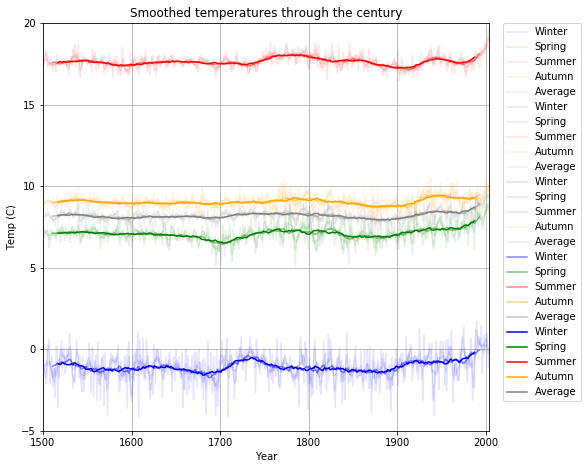This looks almost as intended. The only problem is the abundance of the items in the legend, which is quite normal, since each and every one of our 20 plots (5 of them in 4 different "alpha" versions) has its own label which legend() then collects and displays.

To avoid this, we can define the label to be None for all but the last r:

In :
import matplotlib.pyplot as plt
from math import floor, ceil

# Radii for which to do the smoothing
rs = [ 0, 1, 3, 11, 17 ]

years = list()
djfs = list()
mams = list()
jjas = list()
sons = list()
annuals = list()

fig = plt.figure()
ax = plt.subplot(111)

# There are more Pythonic ways to do this, for example with
# NumPy's transposing or generator expressions, but this is
# more straightforward.
for year,djf,mam,jja,son,annual in seasonal_data():
years.append(year)
djfs.append(djf)
mams.append(mam)
jjas.append(jja)
sons.append(son)
annuals.append(annual)

# Get the smoothed amounts (for each point take the average of
# r values to the left and to the right
for r in rs:
# Remove the first and the last r years as they cannot  be properly smoothed
syears = years[r:-r] if r else years
# Compute the smoothed values
alpha = 0.1 + 0.9*(r/rs[-1])**2 if r else 0.1  # 0 = invisible, 1 = fully visible
plt.plot(syears, smooth(djfs, r), color="blue", alpha=alpha, label="Winter" if r == rs[-1] else None)
plt.plot(syears, smooth(mams, r), color="green", alpha=alpha, label="Spring" if r == rs[-1] else None)
plt.plot(syears, smooth(jjas, r), color="red", alpha=alpha, label="Summer" if r == rs[-1] else None)
plt.plot(syears, smooth(sons, r), color="orange", alpha=alpha, label="Autumn" if r == rs[-1] else None)
plt.plot(syears, smooth(annuals, r), color="gray", alpha=alpha, label="Average" if r == rs[-1] else None)

# Shrink the plot
box = ax.get_position()
ax.set_position([box.x0, box.y0, box.width * 0.8, box.height])

# Define the axes limits
plt.axis([years, years[-1], 5*floor(min(djfs)/5), 5*ceil(max(jjas)/5)])

# Title and axes labels
plt.title("Smoothed temperatures through the century")
plt.xlabel("Year")
plt.ylabel("Temp (C)")

# Display grid
plt.grid()

# Save the plot as a PNG image
plt.savefig("europe-temps-smooth.png", bbox_inches="tight", dpi=200)

# Show the plot
plt.show()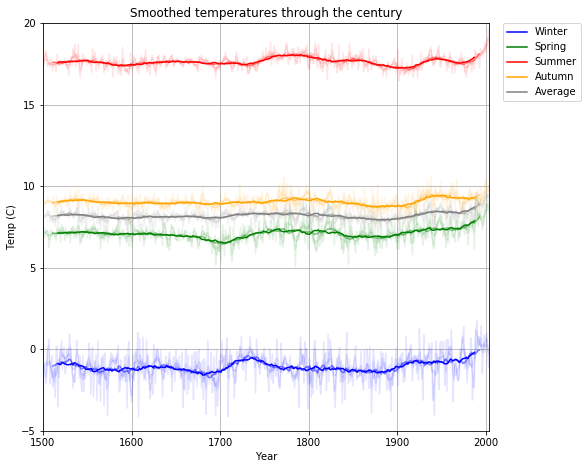And here is our (overly large) saved image, loaded by the IPython-specific function Image:

In :
from IPython.display import Image
Image("europe-temps-smooth.png")
`
Out: